##### Algebra I For Dummies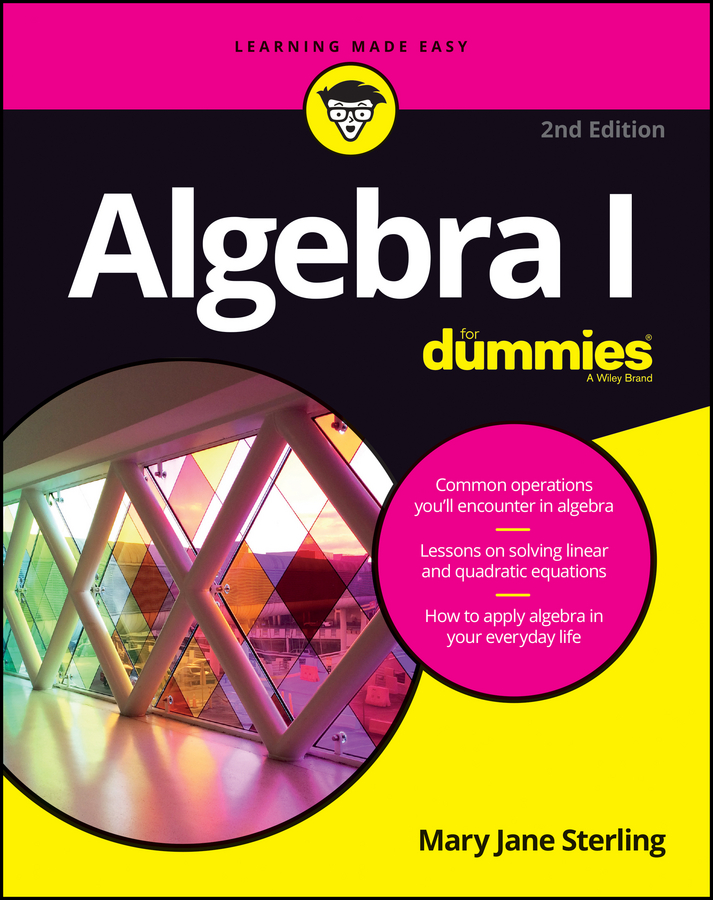So, what is an exponent anyway? According to the Oxford dictionary, an exponent is defined as "a quantity representing the power to which a given number or expression is to be raised, usually expressed as a raised symbol beside the number or expression." Exponents are used in almost all levels of math, from algebra to calculus to physics. Here are two ways you can work with exponents when they show up in formulas and equations.

## How to multiply exponents

You can multiply many exponential expressions together without having to change their form into the big or small numbers they represent. When multiplying exponents, the only requirement is that the bases of the exponential expressions have to be the same. So, you can multiply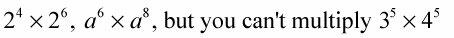because the bases are not the same (although the exponents are).

To multiply powers of the same base, add the exponents together: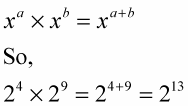If there’s more than one base in an expression with powers, you can combine the numbers with the same bases, find the values, and then write them all together. For example,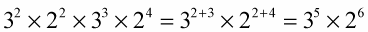Here's an example with a number that has no exponent showing: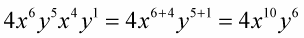When there’s no exponent showing, such as with y, you assume that the exponent is 1, so in the above example, you write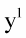## How to divide exponents

You can divide exponential expressions, leaving the answers as exponential expressions, as long as the bases are the same. To divide exponents (or powers) with the same base, subtract the exponents. Division is the opposite of multiplication, so it makes sense that because you add exponents when multiplying numbers with the same base, you subtract the exponents when dividing numbers with the same base.

For example,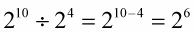Pretty easy, huh? Now wrap your brain around this: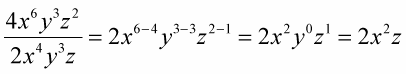Any number to the power of zero equals 1, as long as the base number is not 0.